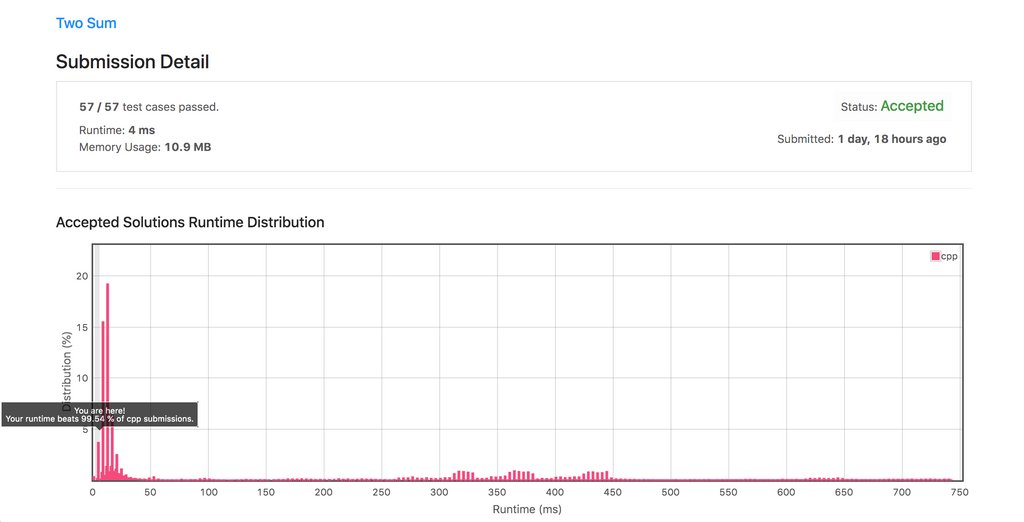Given a list of integers and a target integer, find which pair of values in the list sum to equal the target value and then return the indices of the pair.

## Problem Statement

The Two Sum problem description is below:

Given an array of integers `nums` and an integer `target`, return indices of the two numbers such that they add up to target. You may assume that each input would have exactly one solution, and you may not use the same element twice. You can return the answer in any order.

### First Approach (Brute Force)

Naturally, my first thought was to reach for loops and traverse the array of integers, more specifically the `vector<int>` data structure representing `nums`. Where I would use one outer for loop with a nested for loop. The outer loop would traverse `nums` at O(n) time and for each element A, we will begin traversing `nums` starting from the position of A and begin comparing the sum of each item B with A until we obtain a sum that equals `target`. This nested looping will create O(A * B) runtimes which gives us O(N^2).

O(N^2) runtime (96ms) with 10.1mb memory usage

``class Solution {public:    // O(N*N)=O(N^2) runtime    // An outer loop with nested loop, so we multiply the runtimes O(A * B)    vector<int> twoSum(vector<int>& nums, int target) {        int sum = 0;        int i = 0;        int j = 0;        // Using iterators to traverse `nums`        for (auto it = nums.begin(); it != nums.end(); ++it) {            // Do B for each time you do A            j = i + 1;            for (auto itr = nums.begin() + j; itr != nums.end(); ++itr) {                sum = *it + *itr;                if (sum == target) {                    return {i, j};                }                j++;            }            i++;        };        return {0, 0};    }};``

## One-pass hash table (Fastest Solution)

The algorithm can be improved to run at O(N) by performing a single pass of a hash table. That is, while iterating and inserting elements into the `unordered_map<int, int>`, we check if the current elements complement e.g. `target - nums[i]` already exists in the map. If it does exist, the solution has been found and we return the indices of the pair. The improvement from O(N^2) to O(N) is quite a big improvement, going from a 96ms runtime to 4ms runtime.O(N) runtime (4ms) with 10.9mb memory usage

``class Solution {public:    // O(N) runtime - one-pass through an unordered map    vector<int> twoSum(vector<int>& nums, int target) {        // where Key = nums[i] (the value at each iteration)        // and Value = i (control variable, representing position of item in `nums`)        unordered_map<int, int> umap;                for (int i = 0; i < nums.size(); ++i) {            // search the map            auto it = umap.find(target - nums[i]);                        // Ensure iterator is not pointing            // at the last element outside the container            if (it != umap.end()) {                // search was successful, return indicies                return vector<int> {i, it->second};            }            // populate the map, where the keys are each number            // in `nums` and value is the position            // e.g. control variable `i` as the value            umap[nums[i]] = i;        }        return {0, 0};    }};``